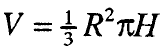# Area and volume of geometric bodies

### 1. AREA AND VOLUME OF A PRISM

The area of a prism is equal to the sum of the areas of its bases B and the area of its shell M, ie.

P = 2B + M

The volume of a prism is equal to the product of the area of the base B and the height of the prism H, ie.

V = B • H

### 2 AREA AND VOLUME OF THE PYRAMID

The area of the pyramid is equal to the sum of the area of the base B and the mantle M, ie.

R = B + M

The volume of any pyramid is equal to one third of the product of the area of the base and the length of its height, ie

### 3. AREA AND VOLUME OF A CUTTING PYRAMID

The area of a truncated pyramid is equal to the sum of the areas of its bases B and B₁ and the sheath M, ie.

Р = В + В₁ + M

If the height of the truncated pyramid is H, and the areas of the bases are B and B₁, then its volume is

### 4. AREA AND CYLINDER VOLUME

The area of a cylinder is equal to the sum of the areas of the base B and the casing M, ie.

P = 2B + M, ie P
= 2πR (R + H)

The volume of any cylinder is equal to the product of the area of its base and height, ie.

### 5. AREA AND VOLUME OF CONE

The area of a cone is equal to the sum of the area of the base B and the sheath M, ie.
R = B + M, ie
R = πR (R + s),

(R – radius of the base, ѕ – generatrix).

The volume of any cone is equal to one third of the product of the area of
the base and its height, ie.### 6. AREA AND VOLUME OF CUTTED CONE

The area of a truncated cone is equal to the sum of the areas of the two bases and the area of its casing, ie.

P = π [ R² + r² + s (R + r)]

The volume of a truncated cone is calculated by the formula:

### 7. BALL VOLUME AND BALL PARTS

The volume V of a ball of radius R is calculated by the formula

The volume V of a ball slice is calculated by the formula

in which R is the radius of the ball, h is the height of the dome.

The volume V of a ball segment with height h and radius of the ball R is calculated by the formula

The volume V of a ball layer with height h and radii of the boundary circles r1 and r2 (Fig.23) is calculated by the formula

###### – area and volume of geometric wire –
Total Page Visits: 1074 - Today Page Visits: 2